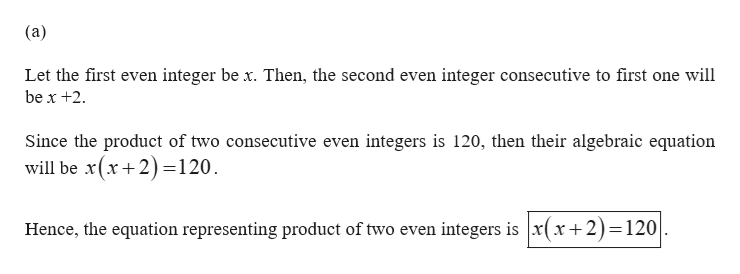# a.Write an equation representing the fact that the product of two consecutive even integers is 120.b.Solve the equation from part (a) to find the two integers.

Question
1 views

a.Write an equation representing the fact that the product of two consecutive even integers is 120.

b.Solve the equation from part (a) to find the two integers.

check_circle

Step 1help_outlineImage Transcriptionclose(a) Let the first even integer be x. Then, the second even integer consecutive to first one will be x +2. Since the product of two consecutive even integers is 120, then their algebraic equation will be x(x+ 2)=120. Hence, the equation representing product of two even integers is x(x+2)=120 fullscreen

### Want to see the full answer?

See Solution

#### Want to see this answer and more?

Solutions are written by subject experts who are available 24/7. Questions are typically answered within 1 hour.*

See Solution
*Response times may vary by subject and question.
Tagged in

### Other